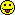## My progress on IUT so far [General Sta­tis­tics]

» » Honestly, I feel like a caveman […]»
» C’mon! Your skills in maths are impressive.

Thanks HelmutI appreciate it a lot.
(*^‿^*)
I'm also grateful that you shared that excerpt (more on this later, because Chapter 7 wasn't available on books.google.com).

Thankfully, I found enough free time to analyze the following IUT theorem using my notes when studying Theorem 8.3.4 from page 122 of this lecture notes. (I don't have access to any of your recommended textbooks yet though).P.S. I intentionally used < instead of ≤ because I feel that in practice, αY will always be less than one. Besides that, my Gaussian level sets didn't scale correctly against my hand-drawn background, so their shapes are compromised; they should actually have the same covariance matrix.

I'm now thinking about all the cases when H0's covariance matrix is different from the true covariance matrix (such as how β would look like) to see how IUT really dealt with dependencies. But a preliminary glance seem to suggest that the above theorem is violated by the following cases, so I definitely need to think deeper on what the theorem actually states (i.e. what it means for IUT to be a level α test of H0 versus H1) to truly understand how the following cases affect the "global" α and β:With that said, now that I am starting to get a feel for IUT, I feel that I am getting closer to truly understand the following facts you shared:»
• Since you have to pass both AUC and Cmax (each tested at $$\alpha$$ 0.05) the intersection-union tests keep the familywise error rate at ≤0.05.• We have three tests. The areas give their type I errors. Since we perform all at the same level, the areas are identical. […] The FWER is given by the area of the intersection which in any case will be ≤ the nominal α.

As of now though, I haven't managed to see the "new" geometric interpretation of correlation (yet), so the following facts are still not within my grasp:

» The FWER gets more conservative the more the PK metrics differ.
» The Euclidean distance between centers give the correlation of PK metrics (here they are identical as well). The FWER is given by the area of the intersection which in any case will be ≤ the nominal α.
»
»In reality the correlation of AUC0–∞ (green) with AUC0–t (blue) is higher than the correlation of both with Cmax (red). If we would test only the AUCs, the FWER would by given again by the intersection which is clearly lower than the individual type I errors. If we add Cmax, the FWER decreases further.

»
»

The proof of the result is almost trivial, at least if one is willing to adopt some piece of the basic formalism customary in expositions of the abstract theory of statistical hypothesis testing methods. […] The condition we have to verify, reads […] as follows:
» $$E_{(\eta_1,\ldots,\eta_q)}(\phi)\leq\alpha\;\textrm{for all}\;(\eta_1,\ldots,\eta_q)\in H\tag{7.3}$$ where $$E_{(\eta_1,\ldots,\eta_q)}(\cdot)$$ denotes the expected value computed under the parameter constellation $$(\eta_1,\ldots,\eta_q)$$. […]»
»     In order to apply the result to multisample equivalence testing problems, let $$\theta_j$$ be the parameter of interest (e.g., the expected value) for the ith distribution under comparison, and require of a pair $$(i,j)$$ of distributions equivalent to each other that the statement $$K_{(i,j)}:\,\rho(\theta_i,\theta_j)<\epsilon,\tag{7.4}$$ holds true with $$\rho(\cdot,\cdot)$$ denoting a suitable measure of distance between parameters. Suppose furthermore that for each $$(i,j)$$ a test $$\phi_{(i,j)}$$ of $$H_{(i,j)}:\,\rho(\theta_i,\theta_j)\geq \epsilon$$ versus $$K_{(i,j)}:\,\rho(\theta_i,\theta_j)< \epsilon$$ is available whose rejection probability is $$\leq \alpha$$ at any point $$(\theta_1,\ldots,\theta_k)$$ in the full parameter space such that $$\rho(\theta_i,\theta_j)\geq \epsilon$$. Then, by the intersection-union principle, deciding in favour of “global quivalence” if and only if equivalence can be established for all $$(_{2}^{k})$$ possible pairs, yields a valid level-$$\alpha$$ test for $$H:\,\underset{i<j}{\max}\{\rho(\theta_i,\theta_j)\}\geq \epsilon\;\textrm{vs.}\;K:\,\underset{i<j}{\max}\{\rho(\theta_i,\theta_j)\}<\epsilon\tag{7.5}$$»

I'm thinking that the excerpt you shared contains the crucial info for me to see the "new" geometric interpretation of correlation, because of the following statement:
» […] with $$\rho(\cdot,\cdot)$$ denoting a suitable measure of distance between parameters.

but I'm very confused by the excerpt's notations, because I couldn't find their corresponding notations in the aforementioned lecture notes (nor by googling); in particular:
【・ヘ・?】
• What is "parameter constellation" $$(\eta_1,\ldots,\eta_q)$$? Because I somehow doubt it corresponds to "parameter space" in my notes (because your excerpt also used the term "parameter space")...I also referred to page 288 of this paper for the definition of "parameter space".

• What is the datatype for H? Because I always thought H0 and H1 were just labels.

• This is my current best guess:• Is your excerpt related to Theorem 8.3.4 (i.e. IUT is a level α test of H0 versus H1) or Theorem 8.3.5 on page 123 (i.e. when will IUT be a size α test of H0 versus H1)?

Thanks in advance for the clarificationNopeThanks for sharing :) Because this is the first time I heard of Anscombe’s quartet, so I found it pretty interesting as a possible example to introduce other statistics (e.g. skew and kurtosis).For some reason though, my mind thought of the Raven Paradox when reading about Anscombe’s quartet. Maybe because they both raised the question on what actually constitutes as evidence for a hypothesis?Ing. Helmut Schütz